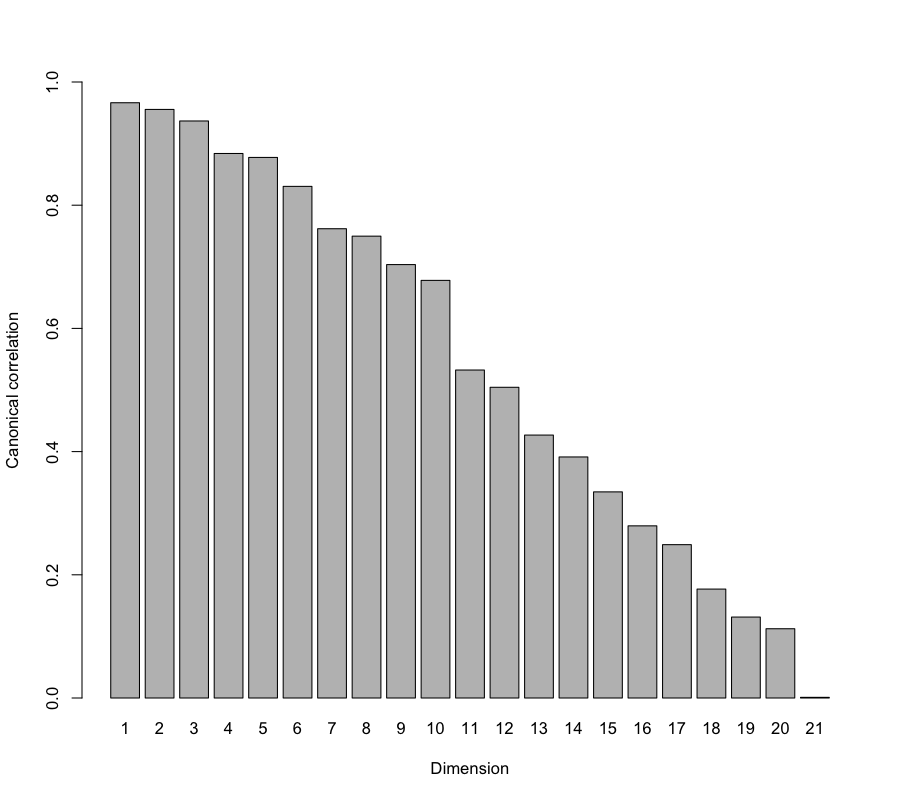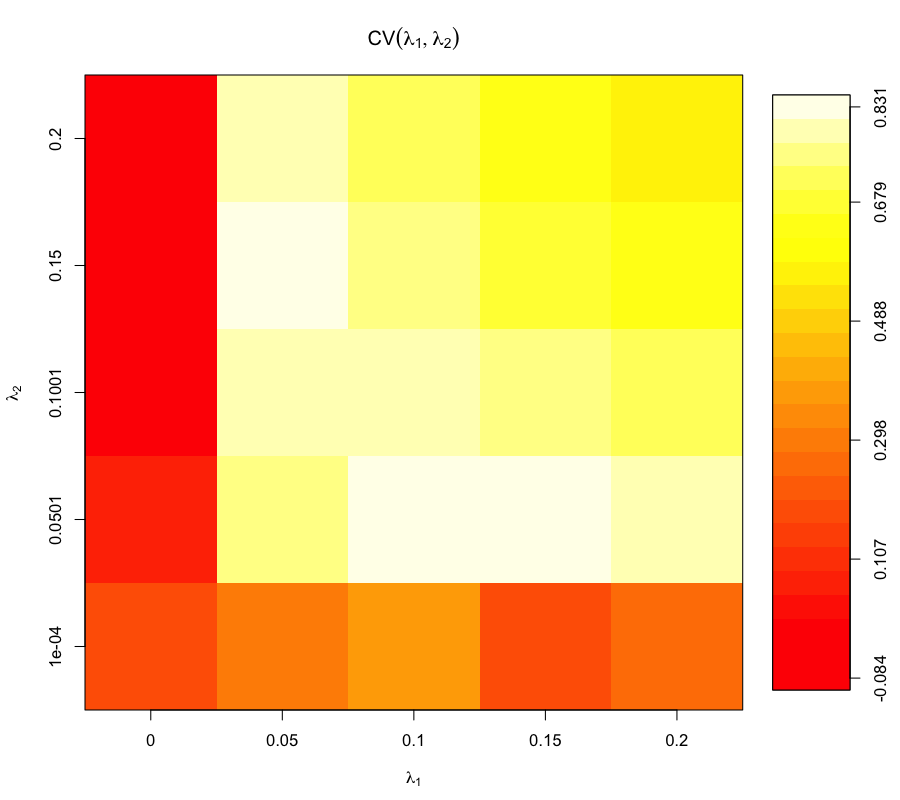# Canonical Correlation Analysis

Canonical Correlation Analysis (CCA) is a multivariate exploratory approach to highlight correlations between two data sets acquired on the same experimental units. In the same vein as PCA, CCA seeks for linear combinations of the variables (called canonical variates) to reduce the dimensions of the data sets, but this time while trying to maximize the correlation between the two variates (the canonical correlation).

Similar to PCA, the user has to choose the number of canonical variates pairs (ncomp) to summarize as much information as possible.

# Regularized Canonical Correlation Analysis (rCCA)

Classical CCA assumes that p < n and q < n, where p and q are the number of variables in each set. In the high dimensional setting usually encountered with biological data, where p + q >> n + 1, CCA cannot be performed:

The greatest canonical correlations are close to 1 as the recovering of canonical subspace does not provide any meaningful information.

We obtain nearly ill-conditioned sample covariance matrices due to the collinearities or near-collinearities in one or both data sets. The computation of the inverses of these sample covariance matrices is unreliable.

Therefore, a regularization step must be included. Such a regularization in this context was first proposed by Vinod (1976), then developed by Leurgans et al. (1993). It consists in the regularization of the empirical covariance matrices of X and Y by adding a multiple of the matrix identity (Id): Cov(X) + λ1Id and Cov(Y) + λ2Id.

In addition to the number of dimensions ncomp to choose, in rCCA, the two parameters to tune are therefore the regularization (or l2 penalties) λ1 and λ2. This is done using cross-validation with the function estim.regul (see below). Note that these two parameters remain unchanged for all dimensions of rCCA. This tuning step may take some computation time.

# Usage in mixOmics

CCA and rCCA are implemented in mixOmics via the function rcc as displayed below.

library(mixOmics)
data(nutrimouse)
X <- nutrimouse$lipid Y <- nutrimouse$gene


## Estimation of penalisation parameters

Before running rCCA, we need to tune the regularization parameters λ1 and λ2. We can use the cross-validation procedure (CV), or the shrinkage method which may output different results. The shrinkage estimate method = “shrinkage” can be used to bypass tune.rcc to choose the shrinkage parameters, see ?rcc.

## Shrinkage Method

nutrimouse.shrink <- rcc(X, Y, ncomp = 3, method = 'shrinkage')


A scree plot can be useful to help choose the number of the rCCA dimensions.

plot(nutrimouse.shrink, scree.type = "barplot")### CV Method

Here we provide an example of estimation of penalisation parameters using the CV method.

## Estimation of penalisation parameters (CV method)
grid1 <- seq(0, 0.2, length = 5)
grid2 <- seq(0.0001, 0.2, length = 5)

cv <- tune.rcc(X, Y, grid1 = grid1, grid2 = grid2, validation = "loo")##   lambda1 =  0.1
##   lambda2 =  0.050075
##  CV-score =  0.8505446

result <- rcc(X, Y, ncomp = 3, lambda1 = cv$opt.lambda1, lambda2 = cv$opt.lambda2)


# Case study

See Case Study: rCCA Nutrimouse for further details and plotting options.

# Reference

1. Leurgans, S.E., Moyeed, R.A. and Silverman, B.W., 1993. Canonical correlation analysis when the data are curves. Journal of the Royal Statistical Society. Series B (Methodological), pp.725-740.

2. [Vinod, H.D., 1976. Canonical ridge and econometrics of joint production. Journal of econometrics, 4(2), pp.147-166.]

3. González, I., Déjean, S., Martin, P.G. and Baccini, A., 2008. CCA: An R package to extend canonical correlation analysis. Journal of Statistical Software, 23(12), pp.1-14.